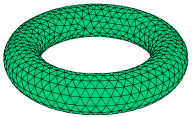﻿ Sample Problems > Usage > 3D_domains > 3d_torus

# 3d_torus

Navigation:  Sample Problems > Usage > 3D_domains >

# 3d_torus{ 3D_TORUS.PDE

This problem constructs a torus.

The top surface and bottom surface meet along the diameter of the torus.

}

title '3D Torus'

coordinates

cartesian3

select

errlim = 0.005

ngrid = 20   { get better mesh resolution of curved surfaces }

painted

variables

u

definitions

Raxis = 4           { the radius of the toroid axis }

Rtube = 1           { the radius of the toroid tube }

{ the torus surface is the locus of points where (Rad-Raxis)^2+Z^2 = Rtube^2 }

 equations    U:  del2(u) + 1 = 0   extrusion   Surface "Bottom" z = -ZTorus   Surface "Top" z = ZTorus   boundaries   surface 1 value(u)=0   surface 2 value(u) = 0   region 1   start(Raxis+Rtube, 0)     value(u) = 0arc(center=0,0) angle=360   { the outer boundary }

start(Raxis-Rtube, 0)

value(u) = 0

arc(center=0,0) angle=360   { the inner boundary }

monitors

grid(x,y,z)

contour(u) on surface z=0

contour(u) on surface y=0

plots

grid(x,y,z)

contour(u) on surface z=0

contour(u) on surface y=0

end